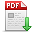# Citation:　Minakshi Ghosh,Manomita Sahu,Dilip Das.Wave Scattering by a Submerged Sphere in Three-Layer Fluid[J].Journal of Marine Science and Application,2022,(1):37-50.[doi:10.1007/s11804-022-00253-2] Click and Copy Wave Scattering by a Submerged Sphere in Three-Layer Fluid## Info

Title:
Wave Scattering by a Submerged Sphere in Three-Layer Fluid
Author(s):
Affilations:
Author(s):
Department of Mathematics, Diamond Harbour Women’s University, Sarisha 743368, India
Keywords:

-
DOI:
10.1007/s11804-022-00253-2
Abstract:
Using linear water wave theory, three-dimensional problems concerning the interaction of waves with spherical structures in a fluid which contains a three-layer fluid consisting of a layer of finite depth bounded above by freshwater of finite depth with free surface and below by an infinite layer of water of greater density are considered. In such a situation time-harmonic waves with a given frequency can propagate with three wavenumbers. The sphere is submerged in either of the three layers. Each problem is reduced to an infinite system of linear equations by employing the method of multipoles and the system of equations is solved numerically by standard technique. The hydrodynamic forces (vertical and horizontal forces) are obtained and depicted graphically against the wavenumber. When the density ratio of the upper and middle layer is made to approximately one, curves for vertical and horizontal forces almost coincide with the corresponding curves for the case of a two-layer fluid with a free surface. This means that in the limit, the density ratio of the upper and middle layer goes to approximately one, the solution agrees with the solution for the case of a two-layer fluid with a free surface.

Memo: